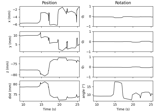# mne.chpi.compute_chpi_amplitudes#

mne.chpi.compute_chpi_amplitudes(raw, t_step_min=0.01, t_window='auto', ext_order=1, tmin=0, tmax=None, verbose=None)[source]#

Compute time-varying cHPI amplitudes.

Parameters
rawinstance of `Raw`

Raw data with cHPI information.

t_step_min`float`

Minimum time step to use.

t_window`float`

Time window to use to estimate the amplitudes, default is 0.2 (200 ms).

ext_order`int`

The external order for SSS-like interfence suppression. The SSS bases are used as projection vectors during fitting.

Changed in version 0.20: Added `ext_order=1` by default, which should improve detection of true HPI signals.

tmin`float`

Start time of the raw data to use in seconds (must be >= 0).

tmax`float`

End time of the raw data to use in seconds (cannot exceed data duration).

verbose

Control verbosity of the logging output. If `None`, use the default verbosity level. See the logging documentation and `mne.verbose()` for details. Should only be passed as a keyword argument.

Returns
chpi_amplitudes`dict`

The time-varying cHPI coil amplitudes, with entries “times”, “proj”, and “slopes”.

Notes

This function will:

1. Get HPI frequencies, HPI status channel, HPI status bits, and digitization order using `_setup_hpi_amplitude_fitting`.

2. Window data using `t_window` (half before and half after `t`) and `t_step_min`.

3. Use a linear model (DC + linear slope + sin + cos terms) to fit sinusoidal amplitudes to MEG channels. It uses SVD to determine the phase/amplitude of the sinusoids.

In “auto” mode, `t_window` will be set to the longer of:

1. Five cycles of the lowest HPI or line frequency.

Ensures that the frequency estimate is stable.

2. The reciprocal of the smallest difference between HPI and line freqs.

Ensures that neighboring frequencies can be disambiguated.

The output is meant to be used with `compute_chpi_locs()`.

New in v0.20.

## Examples using `mne.chpi.compute_chpi_amplitudes`#Extracting and visualizing subject head movement

Extracting and visualizing subject head movement